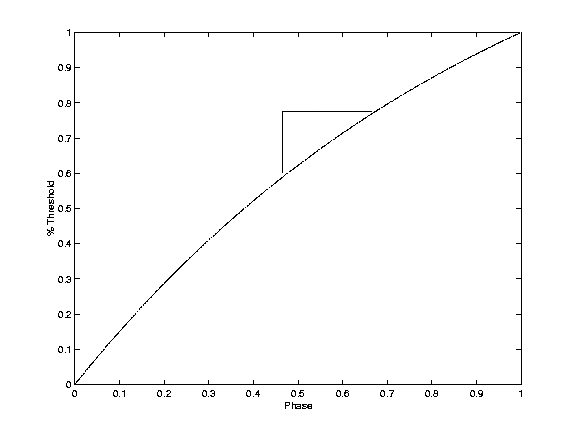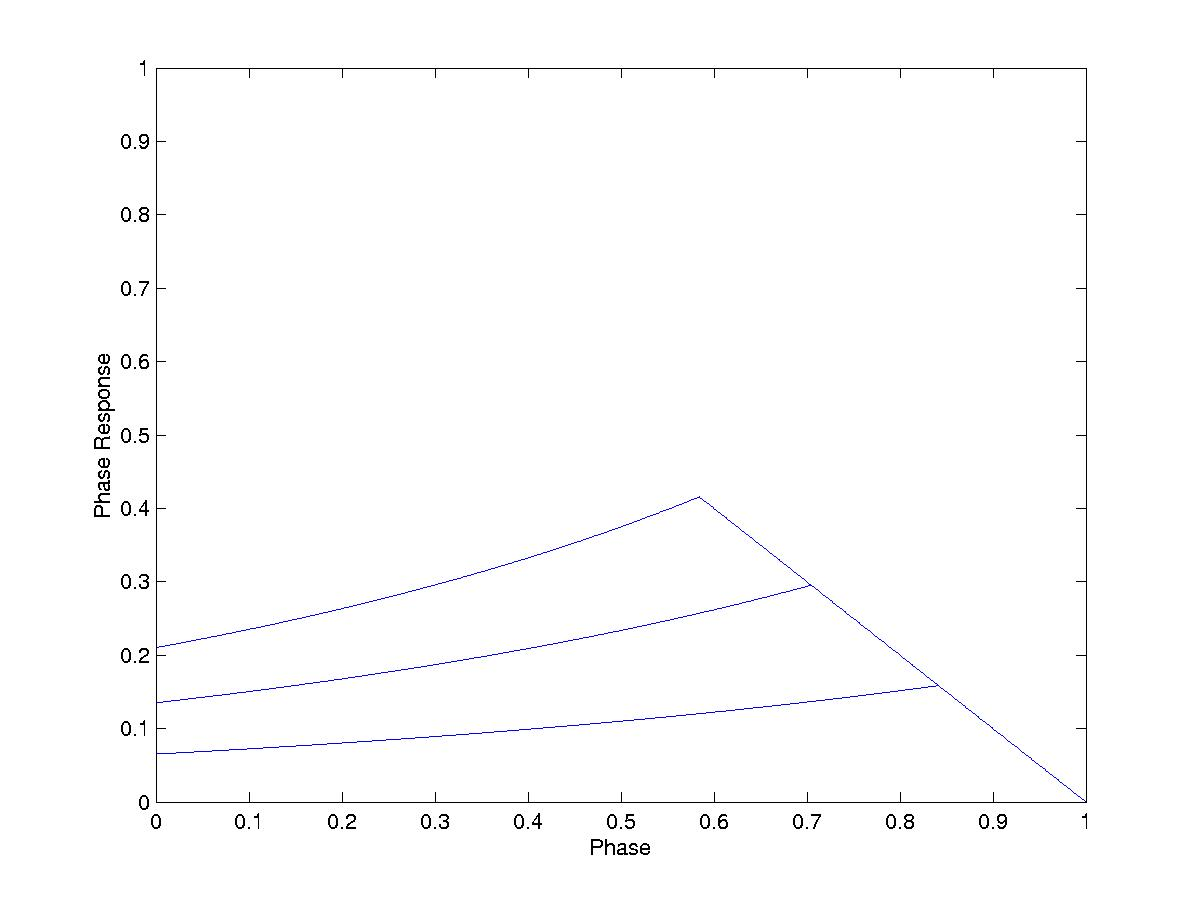## Integrate-and-Fire

The basic concept of integrate-and-fire neurons is that each is at a resting potential (for our purposes 0) and when it hears a spike ("action potential" in neurology terms) its potential increases by a certain amount. When its voltage passes a certain threshold the neuron sends out an action potential to the connected neurons and resets to a reset potential, which is often (but not necessarily) the resting potential. There are two different types of integrate-and-fire neurons: oscillatory and excitatory. Oscillatory neurons approach their threshold (and eventually reach it) even in the absence of inputs (i.e. they have an intrinsic frequency, thus the term "oscillatory"). Excitatory neurons return to the resting potential in the absence of external inputs. [reference here] prove that excitatory neurons can have sustained firing given an initial external input, but this is, for the most part, unimportant but interesting. For obvious reasons, we will only be looking at oscillatory neurons (for obvious reasons).### State Function

Each processor has a state function (the equivalent of a voltage function for neurons), which tells you what it's voltage is at any given point in its phase. The simplest state function is linear. All state functions can be normalized so that the threshold is 1 and the resting state is 0, which turns the state function into a percentage of the threshold (note that this is only a percentage of time till firing if the state function is linear). In systems with identical intrinsic frequencies, the phase can also be normalized onto [0,1]. When a neuron receives a spike, it increases its threshold by the coupling strength epsilon (pictured here as the vertical bar in the middle of the curve), effectively advancing its phase by a certain amount (the horizontal bar in the picture). A typical assumption is that the coupling strength is homogeneous throughout a network. It is quite common that systems will exhibit significantly different behavior for different ranges of the coupling strength. Excitatory coupling is when the coupling strength is positive. Inhibitory coupling is when the coupling strength is negative, in which case the phase is retarded rather than advanced, and often has much more useful and interesting behavior in situations with lag. Since we are engineering a system and not observing one, we may choose both the state function and the coupling strength to elicit the desired behavior. Predicting the behavior caused by a given state function and coupling strength is very difficult, though many different situations have already been analyzed. For the purpose of analysis it is often useful to put restrictions on the state function. For example, Mirollo & Strogatz (1990) used a strictly increasing and concave down function, like the one pictured here.### Phase Response Curve

The state function is then used to define the phase response curve (PRC) of the phase, i.e. the phase by which a node is shifted forward when it hears a spike. The PRCs pictured below are based on the above state function using coupling strengths of .1, .2, and .3. Note that PRCs will be different for different state functions, and will be different (but usually similar, as here) for different coupling strengths (often more dissimilar if you change the sign of the coupling strength). The reason for the downward slope at the end is that the voltage cannot be brought past the threshold. It is often a desirable trait that PRC(0)=PRC(1)=0: i.e. a node does not respond to any incoming spikes when it has just spiked itself. This is not true of the PRC we see here, but in simulation we simply add a check: if the node has fired, it doesn't respond to anything (even though PRC(0) is not 0).

### Peskin's Model

Mirollo & Strogatz (1990) prove that for strictly increasing, concave down state functions with positive coupling strength an all-to-all network will synchronize in finite time1. The model they use, which I have found much success with in lagless situations, is as follows. The specific function I have used in my simulations is a model of a cardiac pacemaker developed by Peskin (1975). dx/dt = S - bx, where S is called the growth and b the dissipation. Mirollo & Strogatz (1990) prove that two nodes will synchronized in time inversely proportional to the coupling strength and the dissipation. For an all-to-all network of more than two nodes, they did not analyze the speed with which it synchronizes, but it is clear from numerical simulation that the more nodes the faster it synchronizes. I usually use S=2, b=1, and varying coupling strength (usually around .3), as Mirollo & Strogatz (1990).

### Analysis

The way in which these nodes synchronize and why they do so is worth noting. (The following is a summary of the analysis in Mirollo & Strogatz (1990).) For two nodes, we say that node A has just fired and is at phase 0, and node B is at phase p. We first define a firing map h(p)=g(f(1-p)+epsilon), where g is the inverse of f (and 1-p is the time it takes for node B to fire). This is visualized in the above picture by the two lines above the curve. After the B fires, we are in the same situation with the roles reversed: node A is at h(p) and node B is at 0. We then define the return map, the phase in which node B ends up after the next time node A fires, as h(h(p)). It is not difficult to show that this map has a single repellant fixed point, p*. If p < p*, then B ends up closer to 0 the next time, and if p > p*, B ends up closer to 1. This means that either A advances B and B retards A, or vice versa. If B ever reaches 0 or 1 (which it does), then A and B have synchronized.

In a network with more than two nodes, the key idea is absorptions. An absorption is when two nodes in the network synchronize. What happens in this network is that there will be one absorption, and then those two nodes are effectively one node with twice the firing strength. Everything is driven into absorbed groups, which are in turn driven together.

1. Actually, they prove that for two nodes there is only one asynchronous stable initial condition, and for more nodes that the set on which the system does not synchronize has Lebesgue measure 0.